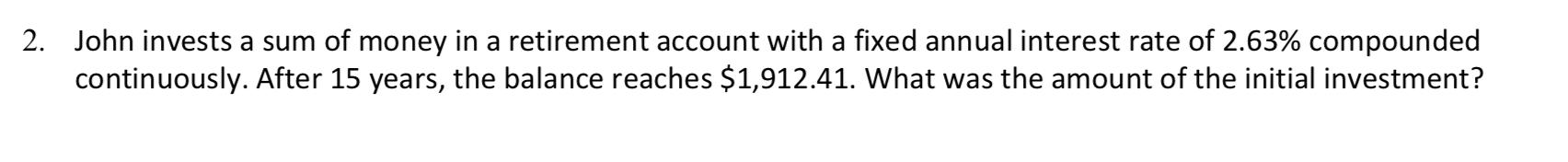# John invests a sum of money in a retirement account with a fixed annual interest rate of 2.63% compoundedcontinuously. After 15 years, the balance reaches \$1,912.41. What was the amount of the initial investment?2.

Question
319 viewshelp_outlineImage TranscriptioncloseJohn invests a sum of money in a retirement account with a fixed annual interest rate of 2.63% compounded continuously. After 15 years, the balance reaches \$1,912.41. What was the amount of the initial investment? 2. fullscreen
check_circle

Step 1

It is given that,

Annual interest rate is 2.63% compounded continuously.

Number of years is 15.

Accumulated value is \$1,912.41.

Step 2

The formula to calculate the present value is given below:

Step 3

Substitute \$1,912.41. for accumulated value, 2.6...

### Want to see the full answer?

See Solution

#### Want to see this answer and more?

Solutions are written by subject experts who are available 24/7. Questions are typically answered within 1 hour.*

See Solution
*Response times may vary by subject and question.
Tagged in